## Line 3 Build Codes.

Build codes run from line 3, 4 and 5. Most of the numbers are the last few digits of the factory production part numbers.

Steering Column: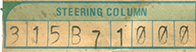The first three digits are the column type:

315 = B-body 4-speed
351 = B-body Column shift Auto Trans
355 = B-body Floor shift Auto Trans

The next two digits are the column color:

B7 = Dark Blue
F8 = Green
X9 = Black

The last four digits are the type of steering:

1000 = manual
1005 = manual A01 light group
7000 = non tilt power steering
7005 = non tilt power steering A01 light group

Steering Wheel: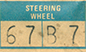The first two digits are the last two digits of the part number of the wheel:

37 = Tuff Wheel
47 = Simulated Wood Wheel

The next two digits are the color:

B7 = Blue
X9 = Black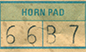The first two digits are the last two digits of the part number of the horn pad:

66 = Simulated Wood horn ring

The next two digits are the color:

B7 = Blue
X9 = Black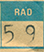The two digits are the last two digits of the part number of the radiator.

61 = 3443961 22" 383 auto
62 = 3443961 22" 383 manual
38 = 3443938 22" 383/440
40 = 3443940 26" 383 A/C
59 = 3443959 26" 383/440/HEMI
60 = 3443960 26" 383/440 A/C

Inlet Hose: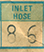The two digits are the last two digits of the part number of the hose.

14 = 3462114
57 = 2863257
86 = 3462186

Alt (Alternator):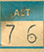The two digits are the last two digits of the part number of the alternator.

10 = 2642610 60amp Single
28 = 2642228 60amp Single
29 = 2642229 60amp Dual
71 = 3438171 30amp Single
72 = 3438172 37amp Single
73 = 3438173 50amp Single
74 = 3438174 60amp Single
76 = 3438176 37amp Dual
77 = 3438177 50amp Dual
78 = 3438178 50amp Dual
78 = 3438378 34amp Single
79 = 3438179 60amp Dual
80 = 3438180 60amp Dual
80 = 3438780 37amp Single
82 = 3438782 50amp Single

FAN: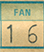The two digits are the last two digits of the part number of the fan.

FAN SPACER: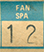The two digits are the last two digits of the part number of the fan spacer or torque drive clutch unit of the fan.

SPACERS

10 = 2120510 1.60
19 = 2863219 1.86
30 = 2120130 2.38
32 = 1825932 1.24
36 = 2265036 2.20
37 = 2265037 .90
46 = 1674746 1.72
55 = 1730155 1.40
56 = 1825956 2.00
59 = 1851959 1.06
60 = 1851960 .70
61 = 1851961 1.30
62 = 3462162 1.93

DRIVES

05 = Thermal 3462105
06 = Thermal 3462106
07 = Thermal 3462107
08 = Thermal 3462108
12 = Torque 3462112
14 = Torque 3769614
16 = Thermal 3769616
17 = Thermal 3769617
18 = Torque 3769618
23 = Thermal 3769623
33 = Torque 2863233
59 = Torque 2863259
60 = Thermal 2863260
61 = Thermal 2863261
63 = Thermal 2863263
64 = Thermal 2863264
66 = Thermal 2863266
67 = Thermal 2863267
68 = Torque 3462168
70 = Torque 2806070
74 = Thermal 3462174
79 = Thermal 3462179
80 = Torque 3462180
82 = Torque 3462182
83 = Torque 3462183

Batteries: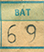The two digits are the last two digits of the factory part number of the battery installed.

20 = 59 amp
51 = 46 amp
64 = 70 amp
66 = 55 amp
67 = 70 amp
69 = 70 amp
81 = 80 amp

V Codes: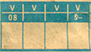First box V0. If it has a 8 in the box it is for delete vinyl roof.

The next two boxes are for V2_ codes, V3_ codes or V4_ codes

V21 = Performance hood paint
V22 = Delete hood paint
V23 = Delete lower body color
V24 = Engine ci Call outs
V20 = Halo Vinyl top

Convertible Top

V3W = White
V3X = Black
V39 = Special Order

Vinyl Canopy Roof

V4W = White
V4X = Black
V4F = Green
V4Y = Gold

The last box V5_ is for Body side molding color

V50 = Chrome
V5B = Blue
V5E = Red
V5F = Green
V5G = Green
V5R = Red
V5W = White
V5X = Black
V5Y = Gold

V Stripe Codes: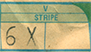A two digit code:
(Note: R/T's and Super Bee's only could have BLACK color or stripe delete.)

V6X = Black longitudinal stripes
V68 = Stripe Delete

Other Stripe codes (Add color code for *)

V4* = Body Stripe
V7* = Accent Stripe
V8* = Transverse Stripe
V9* = Sport stripe